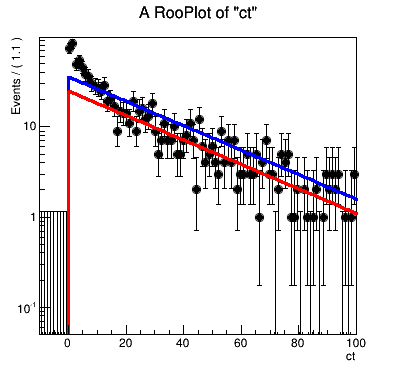# Problem with two RooDecays added together in pyRoot-roofit

UPD Never mind, I solved it myself. That was a bug on my side. ctau1 and ctau0 has to have different names.

Dear RooFitters,
Here is my probelm. I try to add two RooDecays with delta-function resolution and different ctau, generate events from the model and then fit it to the same model. Like so:

``` decay1 = RooDecay('decay1','decay x delta', ct, ctau1, tm, RooDecay.SingleSided) decay0 = RooDecay('decay0','decay x delta', ct, ctau0, tm, RooDecay.SingleSided) sigfrac = RooRealVar("sigfrac","fraction of signal",0.7, 0.69, 0.71) model = RooAddPdf("model","signal(decay1) + bkg(decay0)", decay1, decay0, sigfrac) gen = model.generate(RooArgSet(ct), 1000) model.fitTo(gen) ```

The construction of the model and generation part seem to work fine.
But then, when I fit the toys back to the model, it does not work and seems to fit only to one of the Decay component.